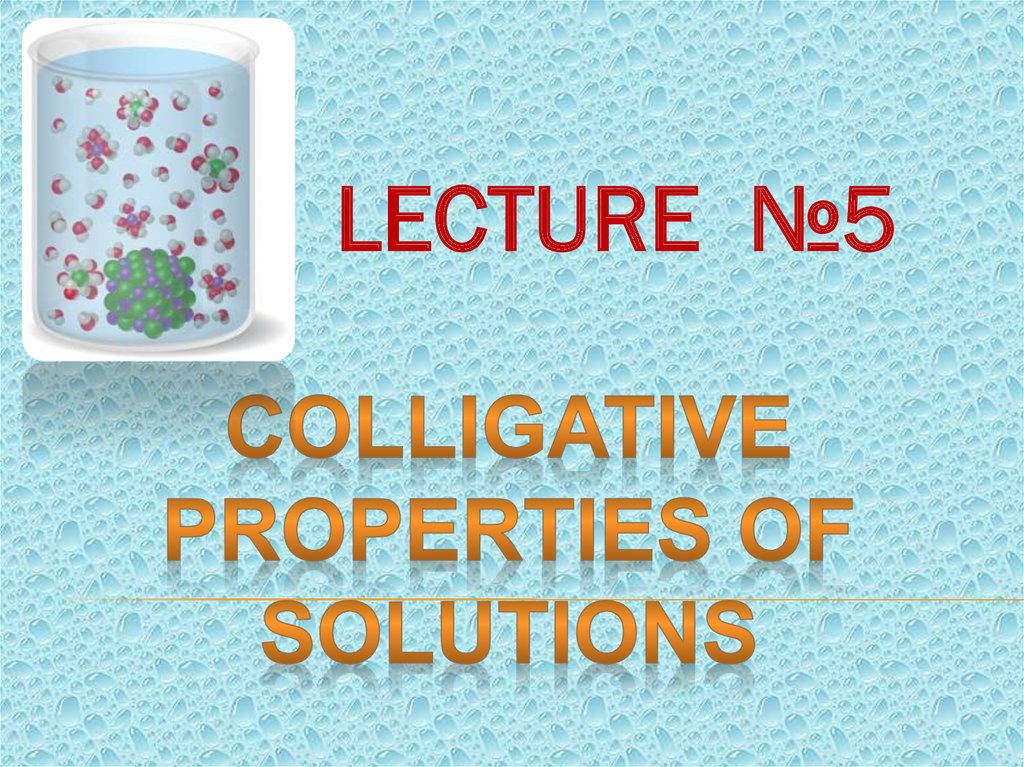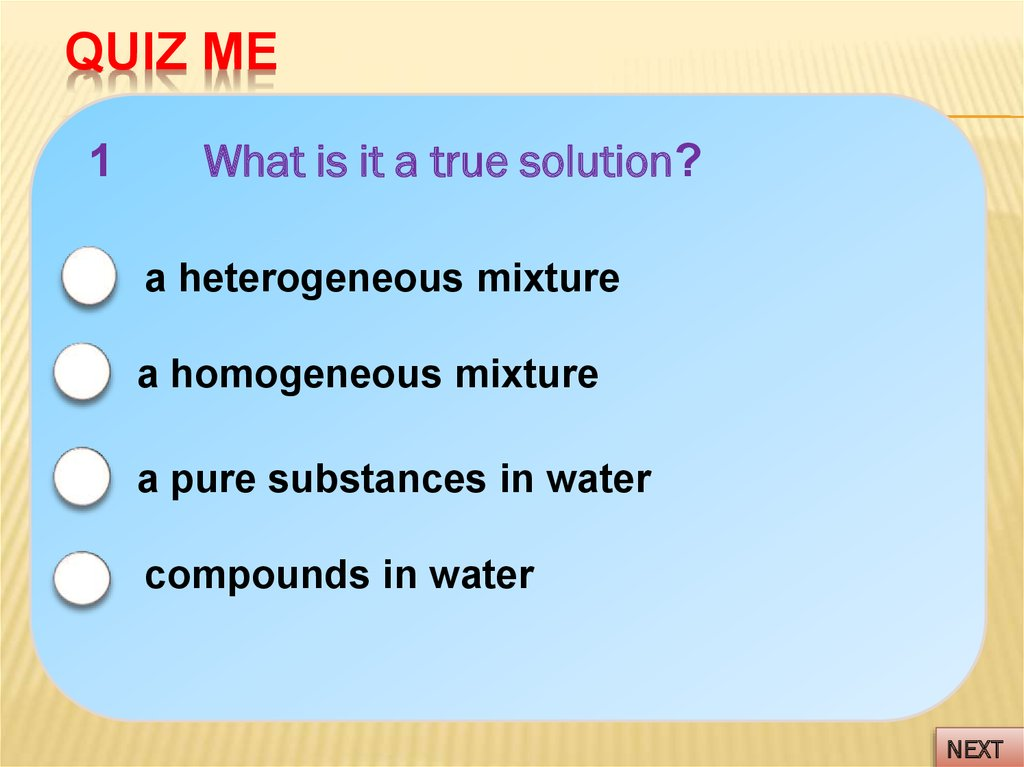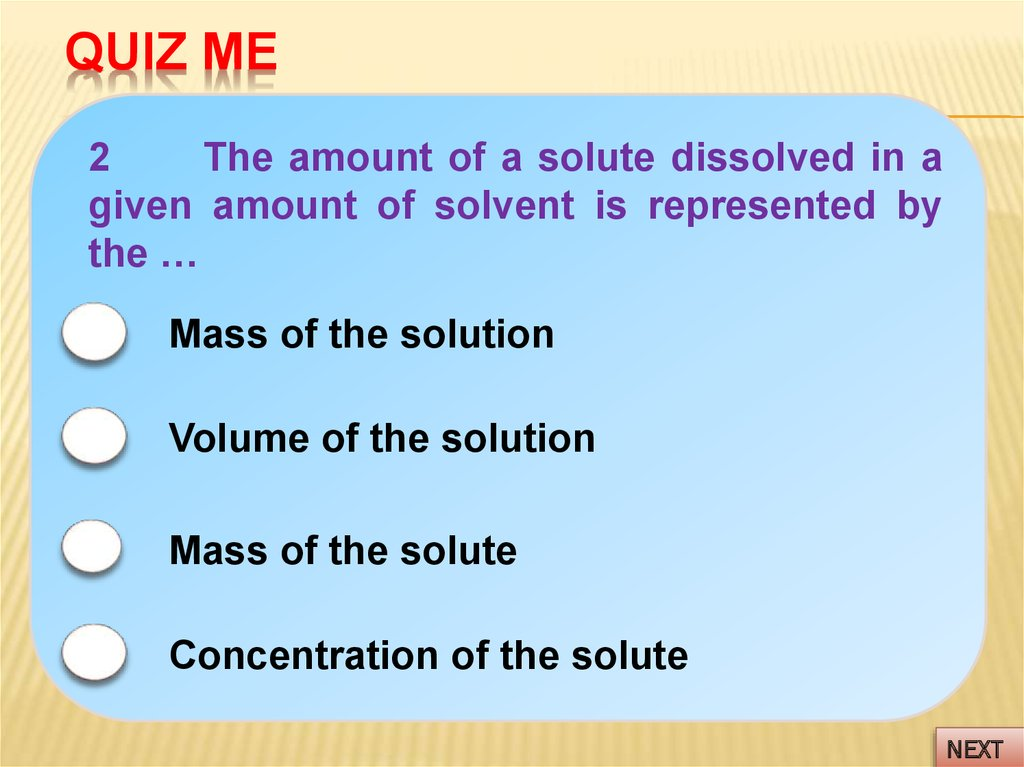# Colligative properties of solutions

LECTURE №5

## 2. QUIZ ME

1
What is it a true solution?
a heterogeneous mixture
a homogeneous mixture
a pure substances in water
compounds in water
NEXT

## 3. QUIZ ME

2
The amount of a solute dissolved in a
given amount of solvent is represented by
the …
Mass of the solution
Volume of the solution
Mass of the solute
Concentration of the solute
NEXT

## 4. QUIZ ME

3
What is the molar concentration of
solution?
the gram of a solute per 1 milliliter of solution
the number of moles of solute per 1 kilogram
of solvent
the number of equivalent-moles of solute
per 1 liter of solution
the number of moles of solute per 1 liter of
solution

## 5. QUIZ ME

4
What is the molality of solution?
the gram of a solute per 1 milliliter of solution
the number of moles of solute per 1 kilogram
of solvent
the number of equivalent-moles of solute
per 1 liter of solution
the number of moles of solute per 1 liter of
solution

## 6.

Nature of Solute
Non-electrolytic are
substances that do
not form ions and
do
not
conduct
electricity
when
placed in water.
An electrolyte is a
substance
that,
when dissolved in
water form ions, as
results a solution
can
conduct
electricity:
NaCl => Na+ + Clcation
anion

## 7.

SOLUTION OF
Electrolyte

solution that conducts
electricity
Nonelectrolyte

solution that does not
conduct electricity
• ionic
compounds
in
polar
solvents dissociate (break apart) in
solution to make ions
• may
be
strong
(100%
dissociation) or weak (less than
100%)
Strong Electrolyte – all or almost
all
of
compound
dissociates;
example:
strong acids (H2SO4,
HNO3, HClO4, HCl, HBr, HI)
Weak Electrolyte – small amount of
compound dissociates; example –
weak acids (HF, CH3COOH, H3PO4)
• solute is dispersed but does not
dissociate
Example: sugar (dissolves but does
not
dissociate),
organic
acids
(contain carboxyl groups)

## 8. Colligative PropertIES

COLLIGATIVE PROPERTIES
Colligative properties are the
set of properties that depend only
on the concentration of solute
particles (ions or molecules) in the
solution and not the type.
In other words, it doesn’t matter if it is salt, sugar,
gasoline, or tennis balls – it will behave the same way!

## 9. Colligative PropertIES:

COLLIGATIVE PROPERTIES:
1) Vapor Pressure Lowering (1st Raoult’s
Law)
Related to boiling point
2) Freezing Point Depression
And Boiling Point Elevation (2nd Raoult’s
Law)
3) Osmotic Pressure (Van’t Hoff’s Law)
Membrane diffusion
The Great Sugar Fountain

## 10.

When ↑t , ↑p0 H2O:
00C – 4,6 mm Hg
200C – 17,4 mm Hg
1000C – 760 mm Hg
P0
The vapor pressure of water (P0) is the
pressure
at
which
water
vapor
is
in
thermodynamic equilibrium with its condensed
state.

## 11.

Vapor pressure depends on various
factors:
• the nature of the liquid,
• the presence of dissolved substances in
the liquid or solid.
According to Raoult's law, the vapor
pressure of a pure liquid or solid is lowered
by the addition of a solute.

## 12. Vapor Pressure Lowering

VAPOR PRESSURE LOWERING
P
Pure water
>
'
P
Aqueous solution of
nonvolatile solute

## 13.

As
solute
to a solution, the
solvent become less
volatile
(=decreased
vapor pressure).
Solute-solvent
interactions contribute
to this effect.
P0
P0

## 14.

The extent to which a nonvolatile solute lowers the
vapor pressure is proportional to its concentration. This was
discovered by French chemist Francois Raoult (1830-1907).
1st Raoult’s law states that for non-electrolyte
solutions, the partial vapor pressure of a solvent over a
solution (P1) is equal to the vapor pressure of the pure
solvent (P0) multiplied by the mole fraction of the solute
(X2). This law is mathematically expressed as:
1
P P
N
X
P
n
N
2
N X 2 the mole fraction
n X1
X
2
n
X
1
the number of dissolving solute moles
n X 2 the number of solvent mole
X
2
n
X
2

## 15.

The temperature at which vapor
pressure is equal to the atmospheric
pressure (p0= pаtm) is called boiling point
The temperature at which vapor
pressure of solvent in its liquid and solid
phase become equal is called freezing
point
at 00C water is freezing,
and at 1000C water is boiling

## 16.

The
nd
2
Raoult’s Law
One consequence of Raoult's law is that the
boiling point of a solution made of a liquid solvent
with a nonvolatile solute is greater than the
boiling point of the pure solvent. The boiling point
of a liquid or is defined as the temperature at which
the vapor pressure of that liquid equals the
atmospheric pressure. For a solution, the vapor
pressure of the solvent is lower at any given
temperature. This pattern applies to the freezing
point of the solution. Therefore, a higher
temperature is required to boil the solution and a
low temperature – to freeze the solution than the
pure solvent.

## 17.

The boiling temperature of non-electrolytes
solution
tboil solution tboil solvent tboil
If solvent is a water : tboil H 2O 100 C
tboil Cm Esvt
E H 2O 0,52 C
Cm
ebulioscopic constant of solvent
s te 1000
msvt
ms te 1000
C % 1000
M s te msvt M s te (100 C %)
ms te 1000 Esvt
M unknown solute
msvt tboil
ebulioscopic method

## 18.

The change in boiling
point is proportional to
the molality of the
solution:
t boil C m E svt
E H 2O 0,52 C
where Esvt is boiling point elevation constant,
equivalent to 0.52 C/m for aqueous solutions.
This means that, for example, 1 mole of sugar
(non-electrolyte) in 1 kilogram of water will increase
the boiling point from 100 C to 100,52 C. And Cm is
the molal concentration of solute.
It is also important to note that Δtboil is a
positive quantity and should be added to the boiling
point of pure solvent (water), which is 100 C.

## 19.

The freezing temperature of non-electrolytes
solution
t freez solution t freez solvent t freez
If solvent is a water : t freez H 2O 0 C
t freez Cm K svt
K H 2O 1,86 C
ms te 1000 K svt
M s te msvt
krioscopic constant of solvent
ms te 1000 Esvt
M anknown solute
msvt t freez
krioscopic
method

## 20.

The change in freezing
point can be found
similarly:
t freez C m K svt
K H 2O 1,86 C
where Ksvt is the freezing point depression constant
equivalent to -1,86 C/m for aqueous solutions.
Again, for example, 1 mole of sugar (nonelectrolyte) in 1 kilogram of water will decrease the
freezing point from 0 C to -1,86 C.
And since we have already established that the
freezing point of the solution is less than that of the pure
solvent, then Δtfreez is a negative quantity and should be
subtracted from the freezing point of pure solvent (water),
which is 0 C.

## 21.

Some common values for Kfreez and Eboil respectively, are:
Solvent
Kfreez
Eboil
Water
1.86
0.512
Acetic acid
3.90
3.07
Benzene
5.12
2.53
Phenol
7.27
3.56

## 22.

In 1784, the French physicist Jean Antoine Nollet
discovered that a pig's bladder filled with a concentrated
solution of alcohol in water expanded when it was immersed in
water. The bladder acted as a semipermeable membrane,
which allowed water molecules to enter the solution, but kept
alcohol molecules from moving in the other direction.
Movement of one component of a solution through a
membrane to dilute the solution is called osmosis, and the
pressure this produces is called the osmotic pressure ( ).
Osmotic pressure can be demonstrated with the
apparatus shown in the figure below. A semipermeable
membrane is tied across the open end of a thistle tube. The
tube is then partially filled with a solution of sugar or alcohol in
water and immersed in a beaker of water. Water will flow into
the tube until the pressure on the column of water due to the
force of gravity balances the osmotic pressure driving water
through the membrane.

## 23.

During osmosis pure
solvent
diffuses
through a membrane
but solutes do not.
Jean-Antoine Nollet first documented
observation of osmosis in 1748.
Osmosis is defined
as the flow of
water/solvent molec
ules
through
a
semipermeable
membrane from a
region of low to
high solute concent
ration, until equilibrium is established.

## 24.

Net
transfer
of
solvent
molecules into the solution until the
hydrostatic pressure equalizes the
solvent flow in both directions.
Because the liquid level for the
solution is higher, there is greater
hydrostatic pressure on the solution
than on the pure solvent .
Osmotic Pressure:
The excess hydrostatic pressure on
the solution compared to the pure
solvent.

h
H O
2
оsm g h

## 26.

(a) A dilute solution of glucose in water is placed in the right arm of a U-tube, and the left
arm is filled to the same height with pure water; a semipermeable membrane separates the
two arms. Because the flow of pure solvent through the membrane from left to right (from
pure water to the solution) is greater than the flow of solvent in the reverse direction, the
level of liquid in the right tube rises. (b) At equilibrium, the pressure differential, equal to the
osmotic pressure of the solution (Πsoln), equalizes the flow rate of solvent in both
directions. (c) Applying an external pressure equal to the osmotic pressure of the original
glucose solution to the liquid in the right arm reverses the flow of solvent and restores the
original situation.

## 27.

The osmotic pressure of a solution is
the pressure required to stop osmosis. The
osmotic pressure of the solution is given by:
Posm СМ R T
СМ
s te
Vs ln(l )
Van’t Hoff’s equation
ms te
M Vs ln(l )
m
P V
R T
M
Mendeleev Clapeyron equation
where CM is the molarity of solution, R the gas constant
(0.0821 L . atm / K . mol), and T the absolute temperature
(in Kelvins).
The osmotic pressure is expressed in atm.

## 28.

QUIZ ME
Molarirty
units
are
most
appropriate in calculating which of the
following?
A) freezingpoint depression
B) vapor pressure
C) boilingpoint evaluation
D) osmotic pressure

## 29. Types of Solutions Based on Solute Concentration…

Hypotonic (lower solute concentration)
solution
Hypertonic (higher solute concentration)
solution
Isotonic solutions are equal in their
concentrations. We say that they are isotonic
other.
to the
to the
solute
to each

## 30.

Non-electrolyte:
1 М sugar solution
Posm non el s ln
Tboil non el s ln
Т freez non el s ln
i
<<
Electrolyte:
1 М NaCl salt solution
Posm el s ln
Tboil el s ln
Т freez el s ln
ClNa+
С12 Н 22О11
NaCl Na Cl
1 mole solute
1 mole of salt gives 2 moles ions
where i is the Van’t Hoff factor, named after Jacobus Henricus
Vant’ Hoff (1852-1911), who won the very first Nobel Prize in
chemistry in 1901 for his work on colligative properties of
solution.
The i factor gives the number of particles per
formula unit of the solute.

## 31.

For Electrolyte Solution:
Posm i C M R T
t boil i C m E svt
t freez i C m K svt
The degree of dissociation is associated with an
isotonic factor by next ratio:
i 1
n 1
i ( n 1) 1
Al 2 ( SO4 ) 3 2 Al
3
3SO
n 2 cation 3 anion 5
2
4

## 32.

QUIZ ME
If equal numbers of moles of each
of the following are dissolved in 1 kg
of distilled water, the one with the
lowest boiling point will be:
A)NaI
B) AlCl3
C) Mg(NO3)2
D) CH3COOH
E) C6H12O6

## 33.

SUMMARY OF FACTS AND CONCEPTS
1. Colligative properties (or collective properties) are
properties that depend only on the number of solute particles in
solution and not on the nature of the solute particles.
2. The four colligative properties of a solution are vapor
pressure, osmotic pressure, boiling point and freezing point.
3. The change in vapor pressure where the solute is less
volatile than the solvent is regulated by Raoult’s law, which
states that the vapor pressure of a solvent over a solution is
equal to the mole fraction of the solvent times the vapor
pressure of pure solvent.
4. The osmotic pressure of a solution is the pressure
required to stop osmosis.
5. The freezing point of the solution is always less than
the freezing point of the pure solvent.

## 34.

7. In electrolyte solutions, the interaction between ions
leads to the formation of ion pairs. The Van’t Hoff factor (i)
provides a measure of the extent of dissociation of electrolytes in
solution.
8. Solutions can be classified as hypertonic, hypotonic or
isotonic depending on the concentration of solute inside and
outside the cell.
9. A hypertonic solution has a higher concentration of
particle than the cell causing the cell to shrink.
10. A hypotonic solution has a lower concentration of
particles than the cell causing the cell to swell.
11. An isotonic solution has the same osmotic strength on
both sides of the semipermeable membrane.
12. Osmosis is the spontaneous net movement
of solvent molecules through a semi-permeable membrane into a
region of higher solute concentration, in the direction that tends to
equalize the solute concentrations on the two sides.